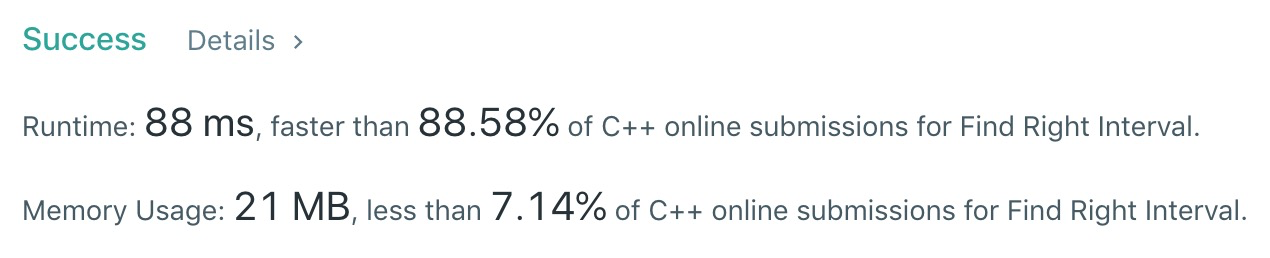# Problem

Given a set of intervals, for each of the interval i, check if there exists an interval j whose start point is bigger than or equal to the end point of the interval i, which can be called that j is on the "right" of i.

For any interval i, you need to store the minimum interval j's index, which means that the interval j has the minimum start point to build the "right" relationship for interval i. If the interval j doesn't exist, store -1 for the interval i. Finally, you need output the stored value of each interval as an array.

Note:

You may assume the interval's end point is always bigger than its start point. You may assume none of these intervals have the same start point.

# Solution

Use brute force. Use a vector “a” as a bitmap to check whether the given number is the lower bound of an interval. If a[i] is -1, the interval with the lower bound of i does not exist, and if a[i] is not -1, the interval with the lower bound of i exists and its index in the given vector “intervals” is a[i].# Code

``````class Solution {
public:
vector<int> findRightInterval(vector<vector<int>>& intervals) {
vector<int> ret;
int size = intervals.size();
int min = 2147483647;
int max = -2147483648;
for (int i = 0; i < size; i++)
{
ret.push_back(-1);
if (intervals[i] > max)
{
max = intervals[i];
}
if (intervals[i] < min)
{
min = intervals[i];
}
}

int len = max - min + 1;
int *a = new int[len];
memset(a, -1, sizeof(int) * len);
for (int i = 0; i < size; i++)
{
a[intervals[i] - min] = i;
}
int j = 0;
for (auto i = 0; i < size; i++)
{
for (j = intervals[i] - min; j < len; j++)
{
if (a[j] != -1)
{
ret[i] = a[j];
break;
}
}
}
return ret;
}
};
``````

504 Love u

echart如何做到多个datazoom时间轴拖动同步

zxmin
2016/11/18
1K
0

2018/01/04
10
0

@Kener-林峰 你好，想跟你请教个问题，我在手机端写的横向柱状图，都是动态获取数据，点击最后一个其他的全部消失，请问这是什么原因呢？ 点击最后一个柱子后，显示 代码： var color = ['rg...

soumns-
2017/06/06
87
1

html： <div style="height:50px;overflow:hidden;font-size: 14px;font-weight: bold;"> <ul class="line"> <li style="line-height:25px;"> <span class="glyphicon glyphicon-hand-right"......

hming
2016/11/28
10
0
Visifire 2.2.0 正式版发布

Visifire 是一个基于SilverLight的 Chart组件，VISIFire 公司提供了 Open Source 的 Silverlight 2 Chart 组件，遵循GPL v3，可以在 ASP, ASP.Net, PHP, JSP, CodeFusion, Ruby on Rails 以及......

2009/05/17
259
0

5
0

7
0
2. 彤哥说netty系列之IO的五种模型

5
0
OSChina 周四乱弹 —— 喵的波粒二象性

Osc乱弹歌单（2019）请戳（这里） 【今日歌曲】 @ 小小编辑推荐：《水墨兰亭》- 李志辉 《水墨兰亭》- 李志辉 手机党少年们想听歌，请使劲儿戳（这里） @巴拉迪维 ：卧室里采光要足够好，这样...

37
1

code-ortaerc

7
0